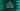# Java program to Find closest number to a given number without a given digit## Java program to find closest number to a Given number without a given digit :

1. This program will find closest number to a user given number that should not contain a user input digit.

2. Result number can be less than or greater than the given number.

3. e.g. Closest number greater than 123 without containing 3 is 124 and smaller than 123 without containing 3 is 122.

4. Using the Scanner class, we will get the number, digit and greater than/less than informations from the user.

5. The digit is scanned as a character.

6. Using a for loop, we will scan all digits one by one.

7. To check if the character is in the digit , we are using Integer.toString(no).indexOf(digit). If the digit is not in the number, it will return -1.

8. Scan one by one and test each number.

### Program :

``````import java.util.Scanner;

public class Main {

public static void main(String[] args) {
Scanner sc = new Scanner(System.in);
int no;
Character digit;
Character c;

System.out.println("Enter a number : ");
no = sc.nextInt();

System.out.println("Enter the digit  : ");
digit = sc.next().charAt(0);

System.out.println("Do you want to look for a number that is larger or smaller than " + no + " ? Please use " +
"\'g\' for greater than or" +
"\'l\' for less than ");

c = sc.next().charAt(0);

if (c == 'g') {
System.out.println("Closest number : " + findClosestNumber(no, digit, true));
} else if (c == 'l') {
System.out.println("Closest number : " + findClosestNumber(no, digit, false));
} else {
}

}

static int findClosestNumber(int no, Character digit, boolean greaterThan) {

if (greaterThan) {
for (int i = no + 1; i > no; i++) {
if (isValidNumber(i, digit)) {
return i;
}
}
} else {

for (int i = no - 1; i > 0; i--) {
if (isValidNumber(i, digit)) {
return i;
}
}
}
return -1;
}

static boolean isValidNumber(int no, Character digit) {
return Integer.toString(no).indexOf(digit) == -1;
}

}
``````

### Examples :

``````Enter a number :
133
Enter the digit  :
1
Do you want to look for a number that is larger or smaller than 133 ? Please use 'g' for greater than or'l' for less than
l
Closest number : 99

Enter a number :
23567
Enter the digit  :
4
Do you want to look for a number that is larger or smaller than 23567 ? Please use 'g' for greater than or'l' for less than
g
Closest number : 23568
``````